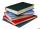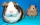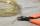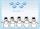Exhibition

The teacher paid for 280 Kč for 4.A students for admission to the exhibition. How many students were on the exhibition?

Result

n =  28

Solution:Leave us a comment of this math problem and its solution (i.e. if it is still somewhat unclear...):Be the first to comment!Next similar examples:

1. Math classificationIn 3A class are 27 students. One-third got a B in math and the rest got A. How many students received a B in math?
2. Foot in busIt was 102 people on the bus. 28 girls had two dogs. A 11 girls had one dog. At the next stop seceded 5 dogs (even with their owners). They got two boys together with three dogs. The bus drove one driver. How many foot were in bus?
3. The resultHow many times I decrease the number 1632 to get the result 24?
4. DoctorsIn the city operates 171 doctors. The city has 128934 citizens. How many citizens are per one doctor?
5. Janka and DankaJanka and Danka began to read the same day the books. Janka had 276 pages, Danka 204 pages. They agreed that both read every day the same number of pages until theirs books read. A) How many maximum pages they had to read the day to honor their agreement?
6. Digit sumDetermine for how many integers greater than 900 and less than 1,001 has digit sum digit of the digit sum number 1.
7. Divisibility 2How many divisors has integer number 13?
8. DivisionWhich number in division 16 give 12 and the rest 3?
9. DivisibilityDetermine all divisors of number 84.
10. Hens and pigsHens and pigs have 46 feet in total. At least how much can heads have?
11. Wire cutA wire of length 7 m was cut into equal lengths using 4 cuts. How long is each piece?
12. PerpetratorThe perpetrator is a number that is smaller than the number 80. It is a multiple of five and it is odd. If we added up the tens and ones of this number we get number 8.
13. Street numbersLada came to aunt. On the way he noticed that the houses on the left side of the street have odd numbers on the right side and even numbers. The street where he lives aunt, there are 5 houses with an even number, which contains at least one digit number 6.
14. Addition of Roman numbersAdded together and write as decimal number: LXVII + MLXIV
15. Roman numeralsWrite numbers written in Roman numerals as decimal.
16. CagesHonza had three cages (black, silver, gold) and three animals (guinea pig, rat and puppy). There was one animal in each cage. The golden cage stood to the left of the black cage. The silver cage stood on the right of the guinea pig cage. The rat was in the
17. Write decimalsWrite in the decimal system the short and advanced form of these numbers: a) four thousand seventy-nine b) five hundred and one thousand six hundred and ten c) nine million twenty-six